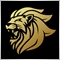# need help finding the highest OrderOpenPrice() to exclude it from OrderModify()37

hello everybody,

if i have more then 1 sell orders opened,

i want to set TakeProfit for all sell orders except the highest one,
any idea how can i do that?
below is my code but it failed aswell ...

```double highest(int op)
{
double c=0;
for (int cnt=0; cnt<OrdersTotal(); cnt++)
{
if(OrderSelect(cnt,SELECT_BY_POS))
if(OrderSymbol()==Symbol() && OrderMagicNumber()== Magic && OrderType()==op)
{
if(OrderOpenPrice()>c)c=OrderOpenPrice();
}
}
return(c);
}
void Modify()
{
double sl=0,tp=0;
for(int cnt=0;cnt<OrdersTotal();cnt++)
{
if(OrderSymbol()==Symbol()&&OrderMagicNumber()==Magic)
if(OrderOpenPrice()==highest(OrderType())&&OrderType()==OP_SELL)
{
tp=0;
}
else if(OrderType()==OP_SELL)
{
tp=OrderOpenPrice()-TakeProfit*Point;
}
{
if(OrderType()==OP_SELL)
{
OrderModify(OrderTicket(),OrderOpenPrice(),sl,tp,0,Red);
}
}
}
}```

Any help Appreciated. thanksModerator
9224

Please don't double post.

```double highest()
{
double openprice=0;

for (int cnt=0; cnt<OrdersTotal(); cnt++)
{
if(OrderSelect(cnt,SELECT_BY_POS))
{
if(OrderSymbol()==Symbol() && OrderMagicNumber()== Magic)
{
if(OrderOpenPrice()>openprice)
{
openprice=OrderOpenPrice();
}
}
}
}
return(openprice);
}```
You just have to add op variable back in.37

Marco vd Heijden:

Please don't double post.

You just have to add op variable back in.

here is my updated code :

```double highest()
{
double openprice=0;

for (int cnt=0; cnt<OrdersTotal(); cnt++)
{
if(OrderSelect(cnt,SELECT_BY_POS))
{
if(OrderSymbol()==Symbol() && OrderMagicNumber()== Magic)
{
if(OrderOpenPrice()>openprice)
{
openprice=OrderOpenPrice();
}
}
}
}
void Modify()
{
double sl=0,tp=0;
for(int cnt=0;cnt<OrdersTotal();cnt++)
{
if(OrderSymbol()==Symbol()&&OrderMagicNumber()==Magic)
if(OrderOpenPrice()==highest(OrderType())&&OrderType()==OP_SELL)
{
tp=0;
}
else if(OrderType()==OP_SELL)
{
tp=OrderOpenPrice()-TakeProfit*Point;
}
{
if(OrderType()==OP_SELL)
{
OrderModify(OrderTicket(),OrderOpenPrice(),sl,tp,0,Red);
}
}
}
}
```

still not working ...Moderator
9224

Missing a bracket.37

Marco vd Heijden:
Missing a bracket.
yes sir this is not the whole code... its only the part i have problem with...
sir the problem is when it open another highest order its not modifying the previous high one you got what i mean?... its working that its providing no takeprofit for the new highest sell opened... but i need it to modify all the others when a new highest sell open you got me?Moderator
9224

Look closer you didn't copy it exactly.

```double highest()
{ 1
double openprice=0;

for (int cnt=0; cnt<OrdersTotal(); cnt++)
{ 2
if(OrderSelect(cnt,SELECT_BY_POS))
{ 3
if(OrderSymbol()==Symbol() && OrderMagicNumber()== Magic)
{ 4
if(OrderOpenPrice()>openprice)
{ 5
openprice=OrderOpenPrice();
} 5
} 4
} 3
} 2
????????
WHERE IS THAT LAST ONE ?????????????????????```Moderator
9224

Hre is my example again:

```double highest()
{// 1
double openprice=0;

for (int cnt=0; cnt<OrdersTotal(); cnt++)
{// 2
if(OrderSelect(cnt,SELECT_BY_POS))
{// 3
if(OrderSymbol()==Symbol() && OrderMagicNumber()== Magic)
{// 4
if(OrderOpenPrice()>openprice)
{// 5
openprice=OrderOpenPrice();
}// 5
}// 4
}// 3
}// 2
return(openprice);
}// 1```

Hmmmm....37

Marco vd Heijden:

Hre is my example again:

Hmmmm....

yes you're right buddy, but even this is not working ,

```double highest()
{
double openprice=0;

for (int cnt=0; cnt<OrdersTotal(); cnt++)
{
if(OrderSelect(cnt,SELECT_BY_POS))
{
if(OrderSymbol()==Symbol() && OrderMagicNumber()== Magic)
{
if(OrderOpenPrice()>openprice)
{
openprice=OrderOpenPrice();
}
}
}
}
return(openprice);
}
void Modify()
{
double sl=0,tp=0;
for(int cnt=0;cnt<OrdersTotal();cnt++)
{
if(OrderSymbol()==Symbol()&&OrderMagicNumber()==Magic)
if(OrderOpenPrice()==highest()&&OrderType()==OP_SELL)
{
tp=0;
}
else if(OrderType()==OP_SELL)
{
tp=OrderOpenPrice()-TakeProfit*Point;
}
{
if(OrderType()==OP_SELL)
{
OrderModify(OrderTicket(),OrderOpenPrice(),sl,tp,0,Red);
}
}
}
}
```369

Then what exactly is not working for you? Describe what you expected vs what you get.Moderator
9224

It's working fine finding the highest order open price over here.37

lippmaje:
Then what exactly is not working for you? Describe what you expected vs what you get.

i need to modify all orders except the highest one,

the modification is to give them a specific takeprofit pips , but leave the highest one with no takeprofit.

so finding the highest sell order and link it to the modifiction function should do well, but its not doing the job,

if a new highest sell order opened, it also leave the others with no takeprofit because they were the highest once,

got what i mean ?